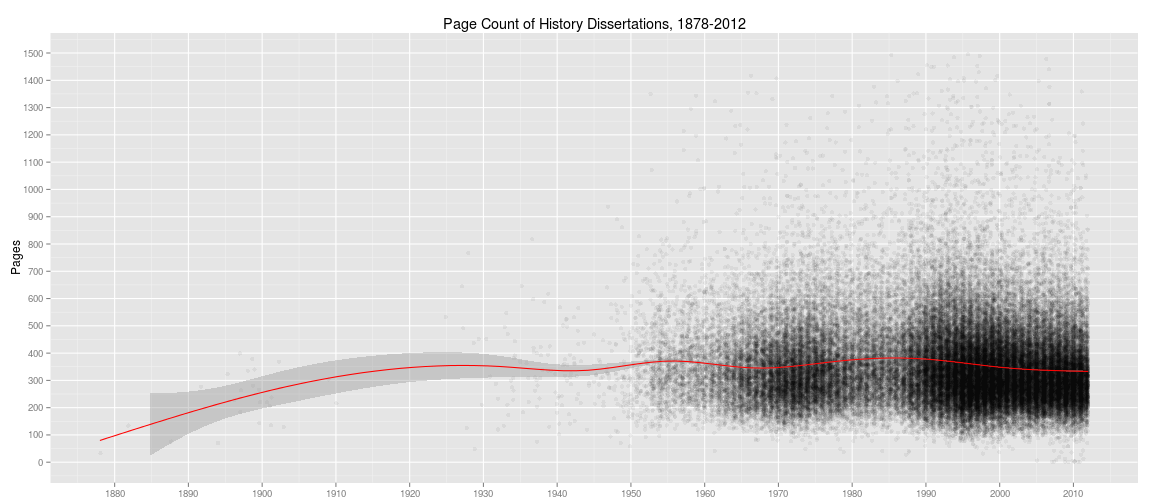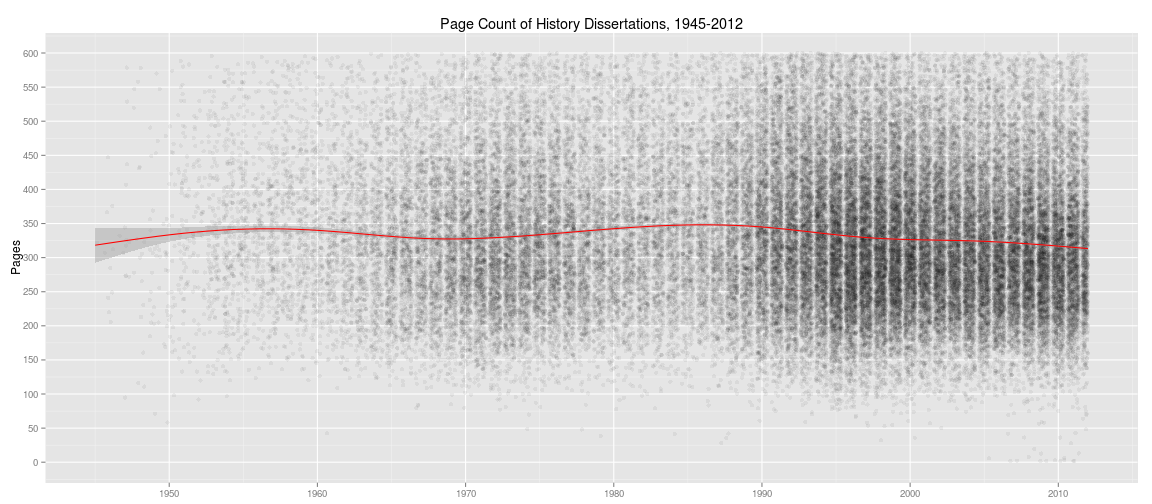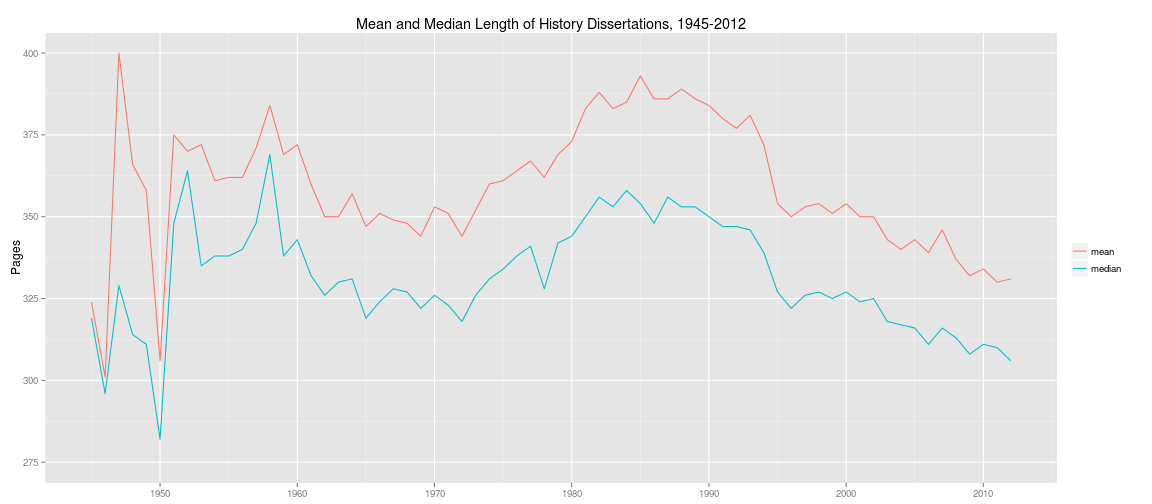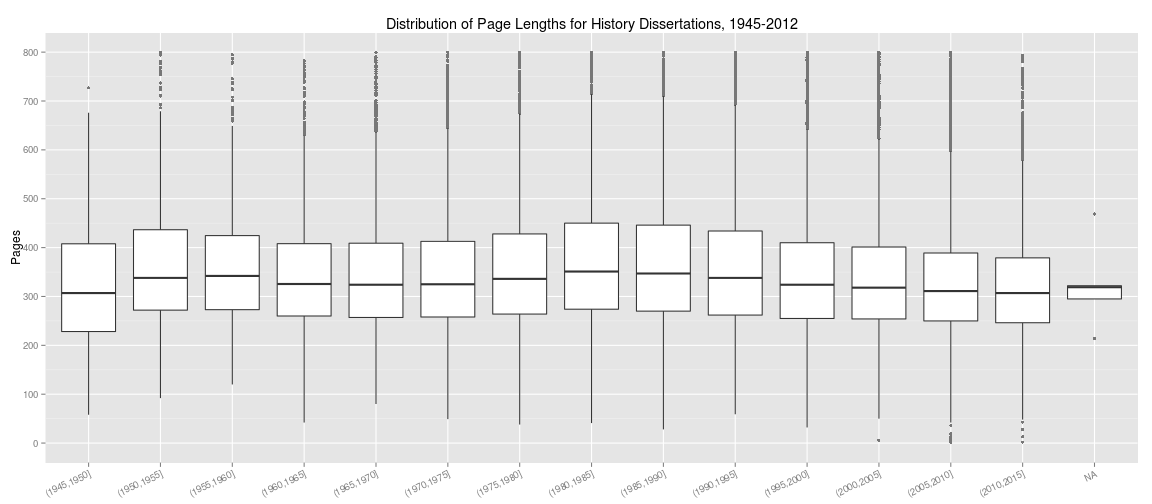# Analyzing Historical History Dissertations: Page Counts

``````library(dplyr)
``````
``````##
## Attaching package: 'dplyr'
##
## The following objects are masked from 'package:stats':
##
##     filter, lag
##
## The following objects are masked from 'package:base':
##
##     intersect, setdiff, setequal, union
``````
``````library(ggplot2)
library(reshape2)
library(knitr)
``````
``````opts_chunk\$set(fig.width = 16, height = 14)
theme_set(theme_gray(base_size = 12))
``````
``````# Some standard scales for chart labels
short_scale <- scale_x_continuous(breaks = seq(1950, 2010, 10), labels = seq(1950,
2010, 10), limits = c(1945, 2012))

long_scale <- scale_x_continuous(breaks = seq(1880, 2010, 10), labels = seq(1880,
2010, 10), limits = c(1878, 2012))

page_scale <- function(min_pages, max_pages, increment) {
scale <- scale_y_continuous(breaks = seq(min_pages, max_pages, increment),
labels = seq(min_pages, max_pages, increment), limits = c(min_pages,
max_pages))
return(scale)
}
``````

The first question anyone writing a dissertation probably asks is, How long should this thing be? When Michael Beck looked at data from the University of Minnesota, he found that history dissertations were the longest. Ben Schmidt found that the average length of history dissertations at Princeton varied quite a bit, from a peak of about 425 pages on average around 1995 to a low of slightly more than 250 pages on average around 2006 or 2007. Ben also concluded that “300 pages is the normal length.”

Using the ProQuest data, we can see how history dissertations varied in length over time:

``````ggplot(h_diss, aes(x = year, y = pages)) + geom_jitter(alpha = 0.05) + geom_smooth(color = "red") +
long_scale + ggtitle("Page Count of History Dissertations, 1878-2012") +
xlab(NULL) + ylab("Pages") + page_scale(0, 1500, 100)
``````
``````## geom_smooth: method="auto" and size of largest group is >=1000, so using gam with formula: y ~ s(x, bs = "cs"). Use 'method = x' to change the smoothing method.
``````
``````## Warning: Removed 2070 rows containing missing values (stat_smooth).
## Warning: Removed 3064 rows containing missing values (geom_point).
``````The more useful view is to look at just dissertations since 1945:

``````ggplot(h_diss, aes(x = year, y = pages)) + geom_jitter(alpha = 0.05) + geom_smooth(color = "red") +
short_scale + ggtitle("Page Count of History Dissertations, 1945-2012") +
xlab(NULL) + ylab("Pages") + page_scale(0, 600, 50)
``````
``````## geom_smooth: method="auto" and size of largest group is >=1000, so using gam with formula: y ~ s(x, bs = "cs"). Use 'method = x' to change the smoothing method.
``````
``````## Warning: Removed 7033 rows containing missing values (stat_smooth).
## Warning: Removed 8018 rows containing missing values (geom_point).
``````We can make a few observations. First, the average length of dissertations is remarkably stable. From 1880 to 1930, history dissertations get quite a bit longer. But since from the 1950s to the present, the average length of dissertations has fluctuated within a relatively narrow band. That band is relatively narrow, that is, in relation to the huge overall variation in the length of history dissertations, which have a normal range between 150 and 600 pages. The acceptable range can even go a little lower than 150 pages, and it can go much, much higher than 600 pages.

We can be more precise about typical length of a history dissertation by plotting the mean and median. (If you prefer, you can see that data in tabular form at the end of the post.)

``````average_pages <- summarise(group_by(h_diss, year), mean = round(mean(pages,
na.rm = TRUE)), median = median(pages, na.rm = TRUE))
average_pages <- arrange(average_pages, year)
average_pages_melted <- melt(average_pages, id = "year")
``````
``````ggplot(average_pages_melted) + geom_line(data = average_pages_melted, aes(x = year,
y = value, color = variable)) + short_scale + page_scale(275, 400, 25) +
labs(color = NULL) + ylab("Pages") + xlab(NULL) + ggtitle("Mean and Median Length of History Dissertations, 1945-2012")
``````
``````## Warning: Removed 72 rows containing missing values (geom_path).
``````The mean length is longer by 27 pages on average than the median length, as you would expect since the permissible maximum length for a dissertation is much more flexible than the permissible minimum length. But the two measures fluctuate more or less in tandem. From a peak in 1958 to a trough in 1972, dissertations got shorter by about 45 pages. Then from 1972 dissertations gradually got longer till they reached a peak in 1988 about 55 pages longer. Since 1988 dissertations are getting shorter, with 2012 being a low with a mean of 331 and a median of 306.

I don't have a good explanation for these fluctuations. Could dissertations have gotten shorter from 1958 to 1972 because of a shift from narrative or political history to social history? Then could they have gotten longer from 1972 to 1988 because of the rise of cultural history? I suppose, though the dates feel vaguely off. What explains why dissertations got shorter through the 1990s and 2000s? I think matching this data up to time-to-degree data and job market data might prove fruitful.

It's not enough to look at the mean or median dissertation length, given that there is such an enormous variation in the permissible length of dissertations. Another helpful way to look at the data is to see the distribution of the quartiles. (This chart cuts off many outliers above 800 pages long.)

``````ggplot(data = filter(h_diss, year >= 1945, year <= 2012), aes(x = cut(year,
pretty(year, 10)), y = pages)) + geom_boxplot(outlier.colour = "#777777",
outlier.size = 1.5) + page_scale(0, 800, 100) + theme(axis.text.x = element_text(angle = 25,
hjust = 1)) + xlab(NULL) + ylab("Pages") + ggtitle("Distribution of Page Lengths for History Dissertations, 1945-2012")
``````
``````## Warning: Removed 2524 rows containing non-finite values (stat_boxplot).
``````The boxes in this chart show the middle 50 percent of dissertations for each half decade. We might interpret this as the typical range for most dissertations. Even typical dissertations fluctuate in length, so that the low end of typical can be 70 pages shorter than median, and the high end of typical can be 50 or 60 pages more than median. But many dissertations come in shorter, and there is a very high upper bound to the maximum length of dissertations.

Next up, I'll compare the typical length of dissertations for the academy as a whole to the length of dissertations at specific departments.

In summary, what does this data about page lengths say about history dissertations? It says that your adviser was right when she said that the dissertation will be done when you've written what you need to write.

``````# Some calculations
short_diss <- filter(h_diss, pages < 100)
short_diss <- arrange(short_diss, pages)

long_diss <- filter(h_diss, pages > 1500)
long_diss <- arrange(long_diss, desc(pages))
``````

Some caveats: There are definitely errors in the data, for example, a six page dissertation from Princeton advised by Robert Darnton. (Sweet deal, if you can get it.) But there are only 215 dissertations with fewer than 100 pages, and only 53 dissertations with greater than 1500 pages, so I don't think these errors skew the data that much. Though it is scarcely believable, the dissertations above 1500 are probably not all errors, either. Another problem is that we're deal g with number of pages rather than word counts, and the number of words per page presumably changes with different writing technologies. (The definition of a word, on the other hand, is stable and timeless, even eternal.) Fortunately the timebound and hideous formatting requirements for dissertations that universitites impose probably keep this variation in check.

#### Mean and Median Length of History Dissertations, 1945-2012

``````kable(filter(average_pages, year >= 1945), format = "pandoc")
``````
``````##   year    mean    median
## ------  ------  --------
##   1945     324       319
##   1946     301       296
##   1947     400       329
##   1948     366       314
##   1949     358       311
##   1950     306       282
##   1951     375       348
##   1952     370       364
##   1953     372       335
##   1954     361       338
##   1955     362       338
##   1956     362       340
##   1957     371       348
##   1958     384       369
##   1959     369       338
##   1960     372       343
##   1961     360       332
##   1962     350       326
##   1963     350       330
##   1964     357       331
##   1965     347       319
##   1966     351       324
##   1967     349       328
##   1968     348       327
##   1969     344       322
##   1970     353       326
##   1971     351       323
##   1972     344       318
##   1973     352       326
##   1974     360       331
##   1975     361       334
##   1976     364       338
##   1977     367       341
##   1978     362       328
##   1979     369       342
##   1980     373       344
##   1981     383       350
##   1982     388       356
##   1983     383       353
##   1984     385       358
##   1985     393       354
##   1986     386       348
##   1987     386       356
##   1988     389       353
##   1989     386       353
##   1990     384       350
##   1991     380       347
##   1992     377       347
##   1993     381       346
##   1994     372       339
##   1995     354       327
##   1996     350       322
##   1997     353       326
##   1998     354       327
##   1999     351       325
##   2000     354       327
##   2001     350       324
##   2002     350       325
##   2003     343       318
##   2004     340       317
##   2005     343       316
##   2006     339       311
##   2007     346       316
##   2008     337       313
##   2009     332       308
##   2010     334       311
##   2011     330       310
##   2012     331       306
##   2013     333       311
``````